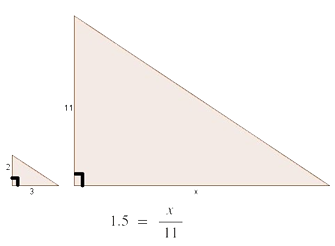Display Accessibility Tools

# Comparing and Scaling

## Topics

Ratios, proportions, rates, scaling ratios and rates, unit rate, constant rate of proportionality, solving proportions, percents, discounts, mark-ups.

## Overview of Changes

Significant Changes Due to the CCSSM introduction of ratio and rates in 6th grade, the content of this unit has been combined into three investigation rather than 4.

## Detailed Description of Changes

CMP2’s Comparing and Scaling has been altered in response to several forces, some internal and some external. There is a cut back (now only 3 investigations) builds on foundational work in 6th grade Units (Comparing Bits & Pieces, Decimal Ops, and Variables & Patterns) and in 7th Grade Unit (Stretching & Shrinking). The internal motivation for change was a desire to more strongly connect to, and to more completely develop, the ideas and strategies initiated in CMP3 Stretching and Shrinking. In similar figures, internal ratios, such as length: width, and external ratios, such as side length X: corresponding side length Y, are constant. When students find a missing length in Stretching and Shrinking, they are solving a proportion; they may use a scale factor rather than set up a proportion, but the proportional reasoning is the same. By the end of CMP3 Stretching and Shrinking students will have a method of scaling a ratio up or down to find a missing quantity or by using equivalent ratios, which is an important strategy for solving any proportion. Learning to choose the scale factor efficiently, in similarity and other situations, is a refinement of this strategy. They also use equivalent ratios to find missing length.

In CMP3 Comparing and Scaling, students use proportional reasoning in contexts other than geometric contexts, and develop additional strategies for solving proportions, including efficient scaling and common denominators. They will see that rate tables are a variation on a scaling strategy, and that unit rates are particularly useful. In the example below, number of units of length of base of triangle: unit length of height of triangle = 1.5: 1. Using this unit rate is another way to solve the proportion 3:2 = x: 11.The external motivations for changing Comparing and Scaling were caused by the requirement, in CCSSM, of the study of ratio in Grade 6, and the addition of constant of proportionality to the content in grade 7. Because students are expected to have some understanding of ratio when they enter grade 7, less time is needed to introduce ratios and rates. Therefore, more time can be spent on developing proportional reasoning and connections among rates, ratios, rate tables and proportions. In addition, since the constant of proportionality implies connections among tables, graphs and equations of proportional relationships, more can be done with these representations. This in turn provides connections to the next unit, Moving Straight Ahead, where students will see that equations of proportional relationships are one kind of linear equation, and the constant of proportionality is, in fact, the slope of the line, or the rate of increase in the table.

New Investigation Changes in CMP2 Investigation
Investigation 1 Ways of Comparing: Ratios and Proportions Investigation 1 contains the CMP2 1.1, 2.1. Problems 1.3 and 1.4 are new. 1.3 focuses on part-part and part-whole with a focus on choosing the appropriate unit of measurement. 1.4 combines many situation that involve proportional reasoning, including one with similar figures. The student need to recognize that all of these situations embody proportionality. The strategies are the same.
Investigation 2 Comparing and Scaling Rates Investigation 2 has three problems with a focus on rates and the constant of proportionality. Problem 2.1 is the same as 2.2 in CMP2. Problems 2.2 and 2.3 are new.
Investigation 3 Markups, Markdowns, and Measures: Using Ratios, Rates, and Proportions Investigation 3 has three problems and the intent is the same as Investigation 4 of CMP2. The percent bar has been introduced in 3.1 as a model for working with percent problems. Problem 3.3 is similar to 2.3 in CMP2. This problem pulls together all of the ideas developed in this unit.